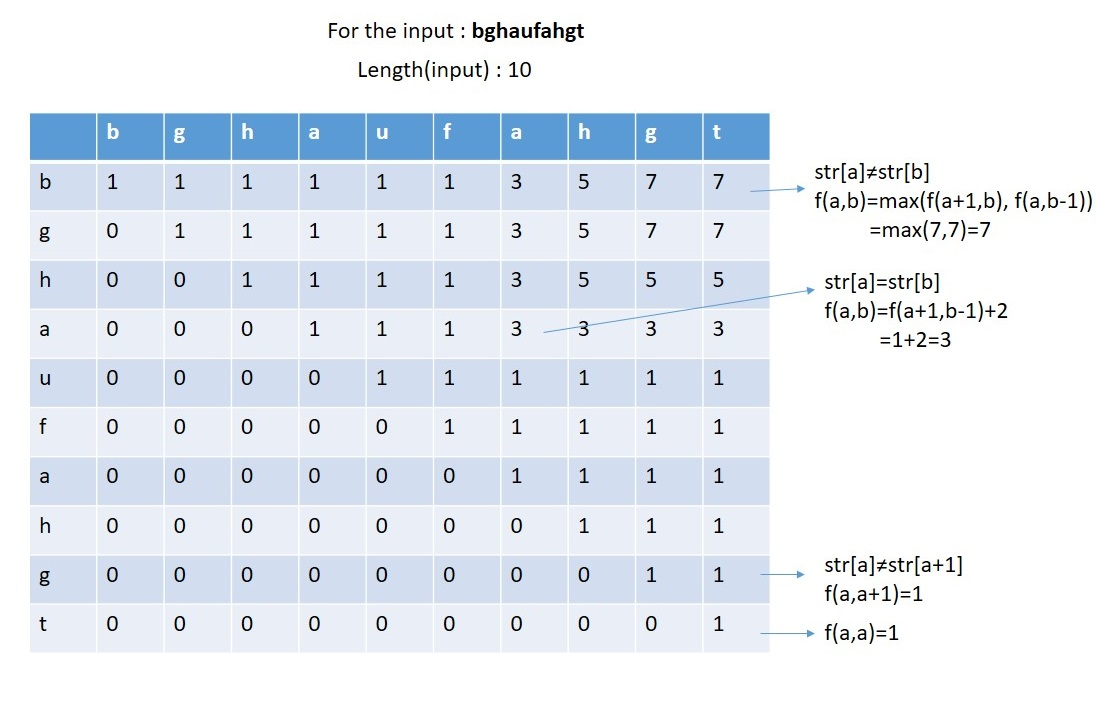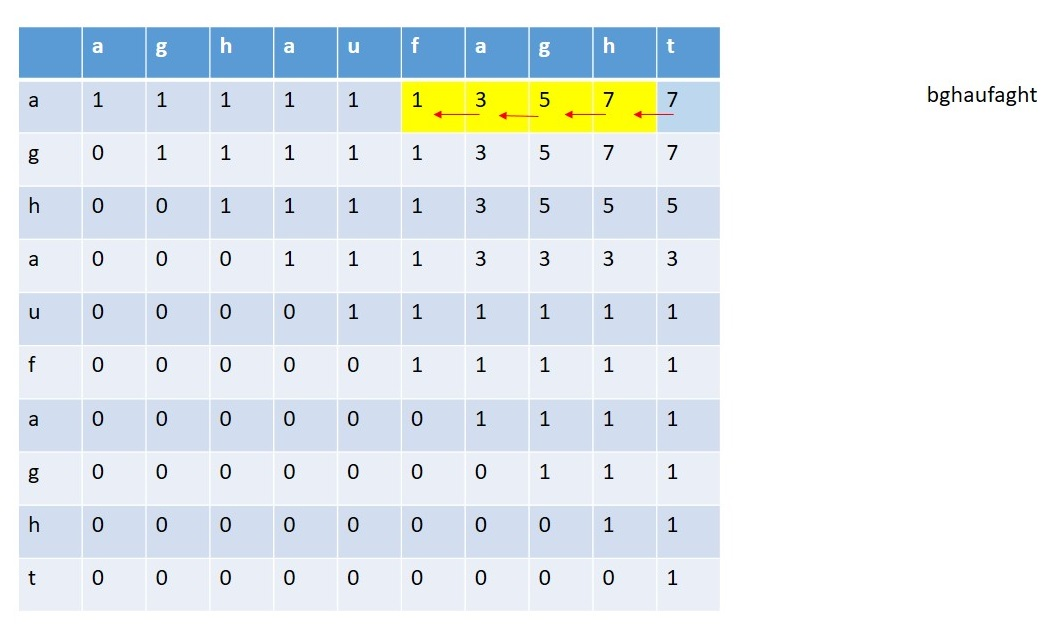# Find out the longest palindromic subsequence from a string

Here, we are going to learn how to find out the longest palindromic subsequence of a string using dynamic programming?
Submitted by Souvik Saha, on March 31, 2020

Problem statement:

Given a string you have to find out the longest palindromic subsequence from the given string.

```    Input:
T Test case
T no of input string will be given to you.

E.g.
3

bghaufahgt
souabbuos
sahajkhahas

Constrain
1≤ length (string) ≤100

Output:
Print the longest palindromic subsequence from that string
```

Example

```    T=3

Input:
bghaufahgt

Output:
ghafahg

Input:
souabbuos

Output:
soubbuos

Input:
sahajkhahas

Output:
sahajahas
```

Explanation with example:

Let there is a string str.

Now possible arrangements are:

1. Single middle characters like aba
2. Paired middle characters like bb

Let, f(a,b) = length of palindromic substring from index a to index b.

Considering the above three facts:

1. If there is only one character then f(a,a) = 1
2. If there are two characters a and a+1 both are same then f(a,a+1) = 2 if both are not same then f(a,a+1) = 1.
3. If there is a substring starting from index a to index b then,
1. If str[a] and str[b] both are same then f(a,b) = f(a+1,b-1) + 2
2. If str[a] and str[b] both are not same then f(a,b)= max{ f(a+1,b) , f(a,b-1) }

For, str = bghaufahgtTo find out the characters in the palindrome we will follow this approach:

1. The max length will be the top right block.
2. Every time we will check the left and bottom adjacent blocks.
1. If both the blocks contain the same value then we will go for any of them.
2. If any block contains a value such that the difference between the current value and the value at the block is 2 then we will go for that block and make a note of that character present in the string whose index is the same as the column number.
3. When we will get the value equals to 1 then stop the traversal.C++ Implementation:

```#include <bits/stdc++.h>
using namespace std;

int arr;

void print_palindrome(string str)
{
int len = str.length();
int i = 0;
int j = len - 1;
int num = arr[i][j];
vector<char> v;
int flag = 0;
while (i < len && j >= 0) {
if (num == 2) {
flag = 1;
break;
}
if (num == 1)
break;
//if the left block has the value by which
//the difference will be 2
if (arr[i][j - 1] + 2 == num) {
v.push_back(str[j]);
j--;
num = arr[i][j];
if (num == 1 || num == 2) {
v.push_back(str[j]);
}
}
//if the bottam block has the value by
//which the difference will be 2
if (arr[i + 1][j] + 2 == num) {
v.push_back(str[i]);
i++;
num = arr[i][j];
if (num == 1 || num == 2) {
v.push_back(str[i]);
}
}
if (arr[i][j - 1] == num) {
j--;
}
else {
i++;
}
}
for (int i = 0; i < v.size(); i++) {
cout << v[i];
}
//cout<<endl;
for (int i = v.size() - 1; i >= 0; i--) {
if (flag && i == v.size() - 1) {
cout << v[i];
}
else if (i != v.size() - 1) {
cout << v[i];
}
}
cout << endl;
}

void length_of_palindrom(string str)
{
int len = str.length();
memset(arr, 0, sizeof(arr));
for (int i = 0; i < len; i++) {
arr[i][i] = 1;
}
for (int i = 0; i < len - 1; i++) {
if (str[i] == str[i + 1])
arr[i][i + 1] = 2;
else
arr[i][i + 1] = 1;
}
for (int l = 3; l <= len; l++) {
for (int i = 0; i <= len - l; i++) {
int j = i + l - 1;
if (str[i] == str[j])
arr[i][j] = arr[i + 1][j - 1] + 2;
else
arr[i][j] = max(arr[i + 1][j], arr[i][j - 1]);
}
}
cout << "Palindrome : ";
print_palindrome(str);
cout << endl;
return;
}

int main()
{
int t;
cout << "Test Case : ";
cin >> t;
while (t--) {
cout << "Enter the string : ";
string str;
cin >> str;
length_of_palindrom(str);
}
}
```

Output

```Test Case : 3
Enter the string : bghaufahgt
Palindrome : ghafahg

Enter the string : souabbuos
Palindrome : soubbuos

Enter the string : sahajkhahas
Palindrome :  sahajahas
```